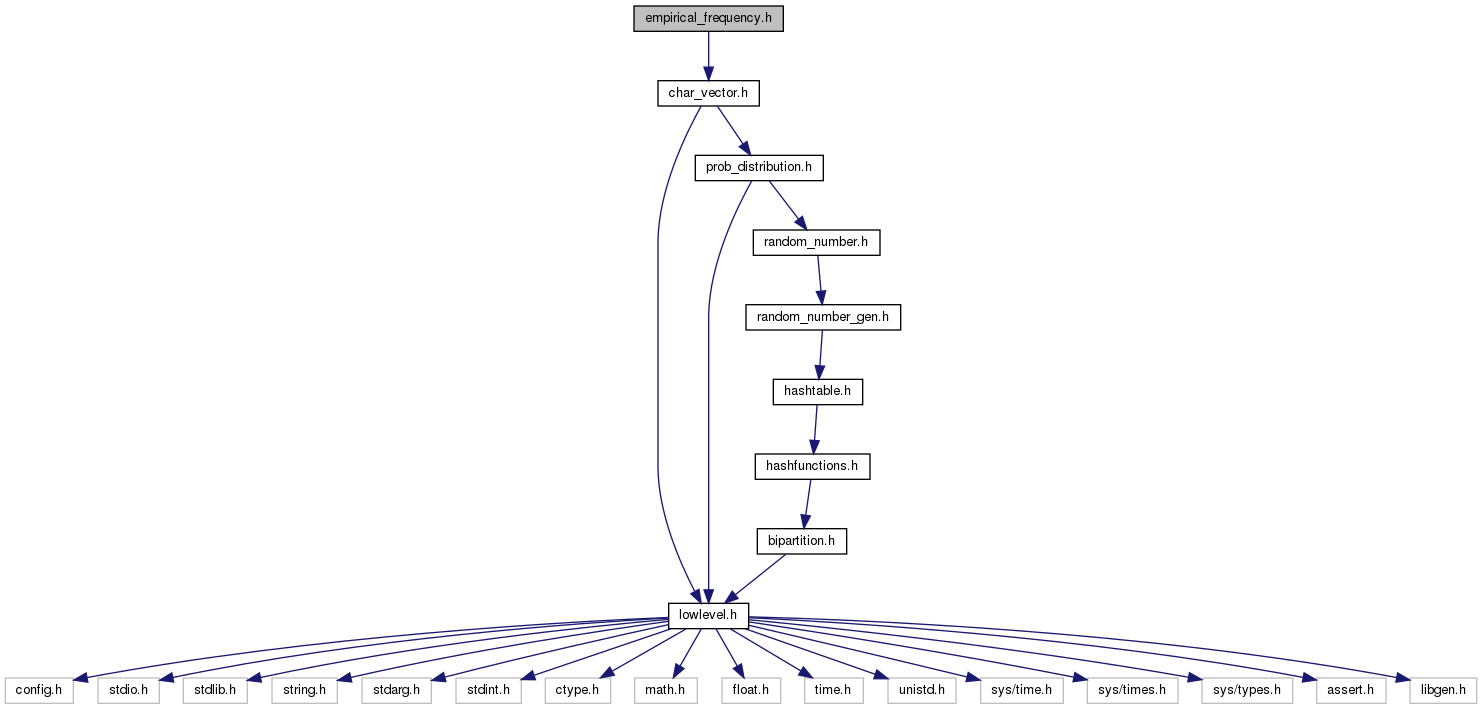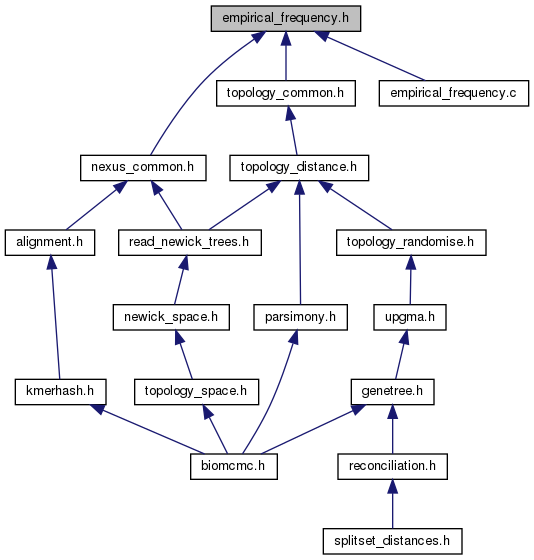biomcmc-lib  0.1 low level library for phylogenetic analysis
empirical_frequency.h File Reference

Creates a histogram of a vector, ordered by frequency. More...

`#include "char_vector.h"`
Include dependency graph for empirical_frequency.h:This graph shows which files directly or indirectly include this file:Go to the source code of this file.

## Data Structures

struct  empfreq_element

struct  empfreq_double_element

struct  empfreq_struct

struct  empfreq_double_struct

## Typedefs

typedef struct empfreq_structempfreq

typedef struct empfreq_double_structempfreq_double

## Functions

void sort_empfreq_decreasing (empfreq ef)

void sort_empfreq_increasing (empfreq ef)

void sort_empfreq_double_decreasing (empfreq_double efd)

void sort_empfreq_double_increasing (empfreq_double efd)

empfreq new_empfreq (int n_elements)

void del_empfreq (empfreq ef)

empfreq_double new_empfreq_double (int n_elements)

void del_empfreq_double (empfreq_double efd)

empfreq new_empfreq_sort_decreasing (void *vec, int n, char type)

empfreq new_empfreq_sort_increasing (void *vec, int n, char type)

empfreq_double new_empfreq_double_sort_decreasing (double *vec, int n)

empfreq_double new_empfreq_double_sort_increasing (double *vec, int n)

empfreq new_empfreq_from_int (int *vec, int n)

empfreq new_empfreq_from_int_weighted (int *vec, int n, int *weight)

int find_mode_int (int *vec, int n)

int find_mode_int_weighted (int *vec, int n, int *weight)

## Detailed Description

Creates a histogram of a vector, ordered by frequency.

Sorts a vector of integers by their frequencies, preserving their original indexes. It is a simple extension to qsort where the original order can be reconstructed, or still a key/value sorting.